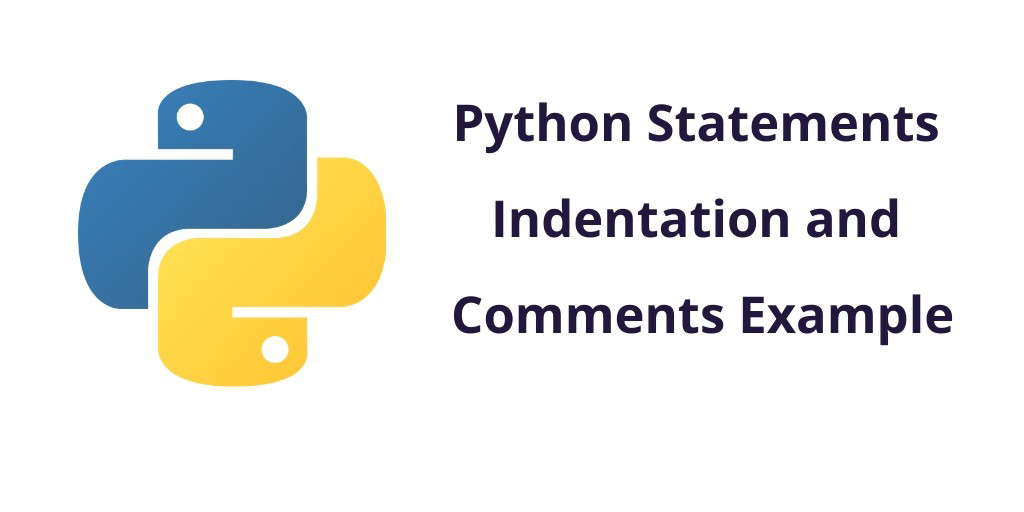Python 30-May-2022

### Python Statements Indentation and Comments Example

This tutorial, you will learn python statements, indentation and comments with example. You will learn why indentation is important in python programming, why use of comments in python programming.

## Python Statements Indentation and Comments Example

### Python Statement

In python programming, A statement is a logical instruction. Which can read and execute by Python interpreter. In Python, it could be an expression or an assignment statement.

#### Simple Assignment Statement

In a simple assignment statement, Here we will create new variables and assign values. You can see the following example below:

#### Syntax

`variable = expression`

For Example:

Let’s take the most basic simple assignment in Python programming.

>>> lang = "Learn Python"

#### Multi-line statement

Generally, in python break the statement line is by a newline character. However, we can make a statement extend over multiple lines with the line continuation character ().

For Example:

x = 5 + 4 + 2 + \
1 + 6 + 4 + \
9 + 7 + 8

Implicit line continuation in python. When you split a statement using either of parentheses ( ), brackets [ ] and braces { }. You need to enclose the target statement using the mentioned construct.

For example

x = (5 + 4 + 2 +
1 + 6 + 4 +
9 + 7 + 8)

For example

colors = ['red',
'blue',
'green']

If you want to write multiple line statements in signle line. So you can use semicolons for that.

For example

a = 1; b = 2; c = 3

### Python Indentation

C, C++, C#, Java high-level programming languages are used braces { } to mark a block of code. But Python uses indentation.

A code block starts with indentation and ends with the first unindented line. And a code block that represents the body of a function, loop, etc.

For example:

for i in range(1,9):
print(i)
if i == 5:
break

Comment writing is a good programming practice and it’s very important part of programming. They are non-executable chunks of line, yet are quite necessary in a program. These not only help other programmers working on the same project. It describes what is happening inside a program so that the person looking at the source code does not have a hard time removing it. but testers can also refer to them for clarity on white-box testing.

In Python, you can use (#) symbol to start writing a comment.

#### Single line comment

You can write single line comments using # in Python.

For Example:

#This is hello world by tutsmake.com
print('Hello world')

#### Multi line comment

Also, You can write multi-line comments using # in Python.

For Example:

#This is hello world program
#by tutsmake.com
#hello
print('Hello world')

Second way of doing multi line comments in python programs, so you can use triple quotes, either ”’ or “””.

These triple quotes are generally used for multi-line strings. But they can be used as multi-line comment as well.

For example

"""This is also a
multi line
print('Hello world')

#### Docstring in Python

Docstring is short for documentation string.

Comments and docstrings give values to a program. They make your programs more readable and maintainable. Even if you need to refactor the same code later, then it would be easier to do with comments available.

Triple quotes are used while writing docstrings.

For example:

def myFunc(no):
"""Function to 10x the value"""
return 10*no

You can access code above using the following special attribute __doc__ of the function.

>>> print(myFunc.__doc__)